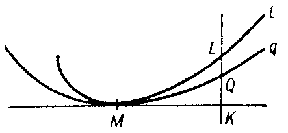# Osculation

of a curve $q$ with a curve $l$ at a given point $M$
A geometrical concept, meaning that $q$ has contact of maximal order with $l$ at $M$ in comparison with any curve in some given family of curves $\{q\}$ including $q$. The order of contact of $q$ and $l$ is said to be equal to $n$ if the segment $QL$ is a variable of $(n+1)$-st order of smallness with respect to $MK$ (see Fig., where $QL$ is perpendicular to the common tangent of $q$ and $l$ at $M$).Thus, of all the curves in $\{q\}$, the curve having osculation with $l$ is the one which is most closely adjacent to $l$ (that is, for which $QL$ has maximal order of smallness). The curve in $\{q\}$ having osculation with $l$ at a given point $M$ is called the osculating curve of the given family at this point. E.g., the osculating circle of $l$ at $M$ is the circle having maximal order of contact with $l$ at $M$ in comparison with any other circle.
Similarly one can define the concept of osculation of a surface $S$ in a given family of surfaces $\{S\}$ with a curve $l$ (or with a surface) at some point $M$ of it. Here the order of contact is defined similarly, except that one must examine the tangent plane of $S$ at $M$ instead of the tangent line $MK$ in the figure.